# 3 DERIVATIVES DERIVATIVES 3 2 The Derivative as

• Slides: 62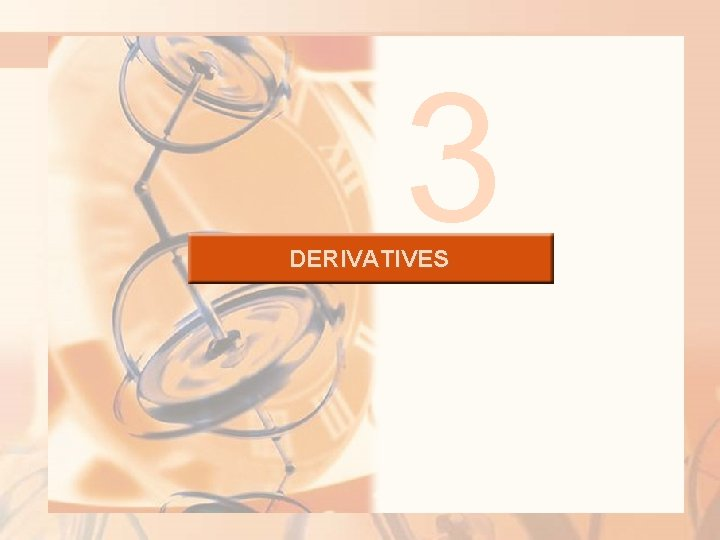3 DERIVATIVES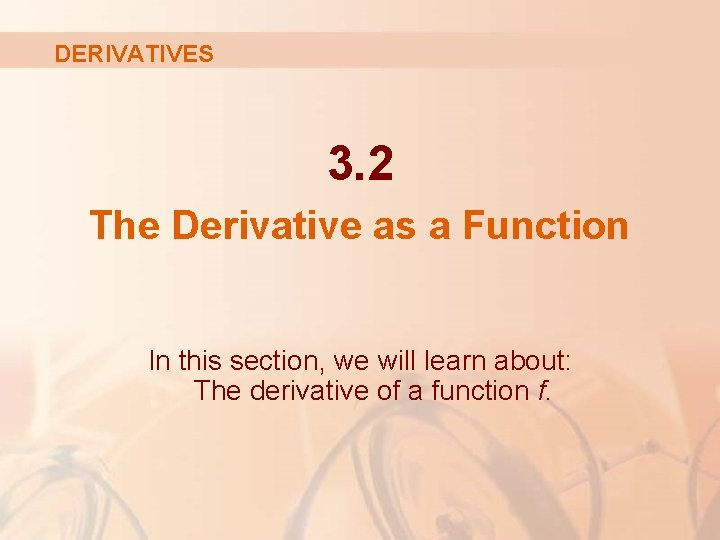DERIVATIVES 3. 2 The Derivative as a Function In this section, we will learn about: The derivative of a function f.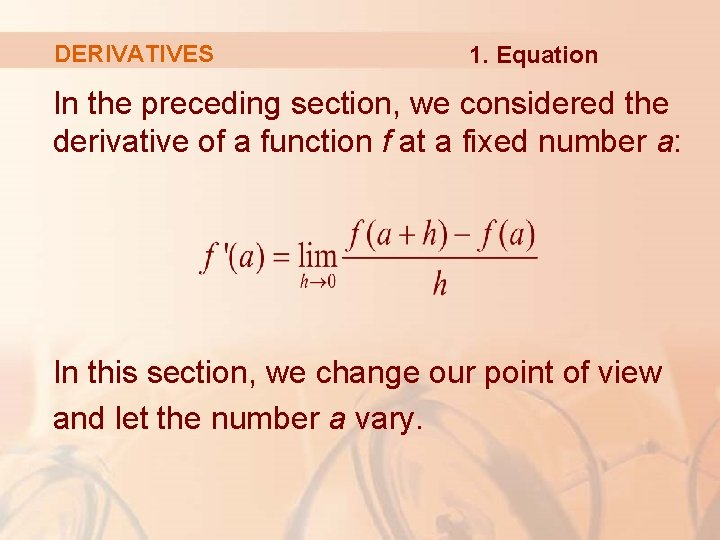DERIVATIVES 1. Equation In the preceding section, we considered the derivative of a function f at a fixed number a: In this section, we change our point of view and let the number a vary.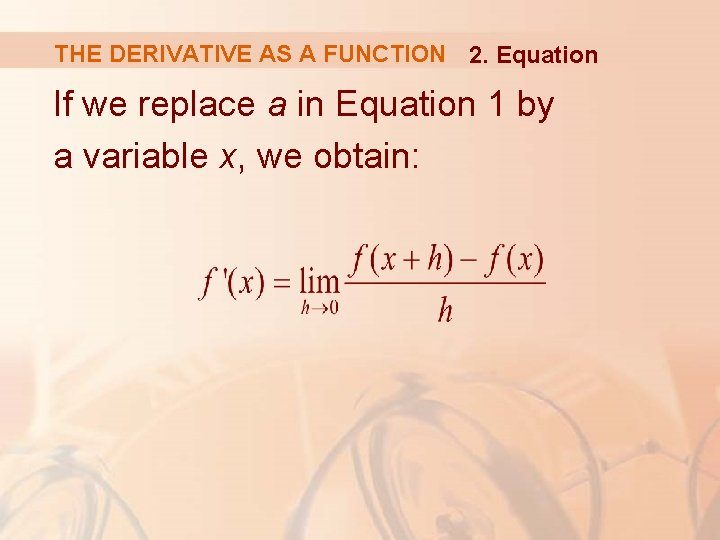THE DERIVATIVE AS A FUNCTION 2. Equation If we replace a in Equation 1 by a variable x, we obtain: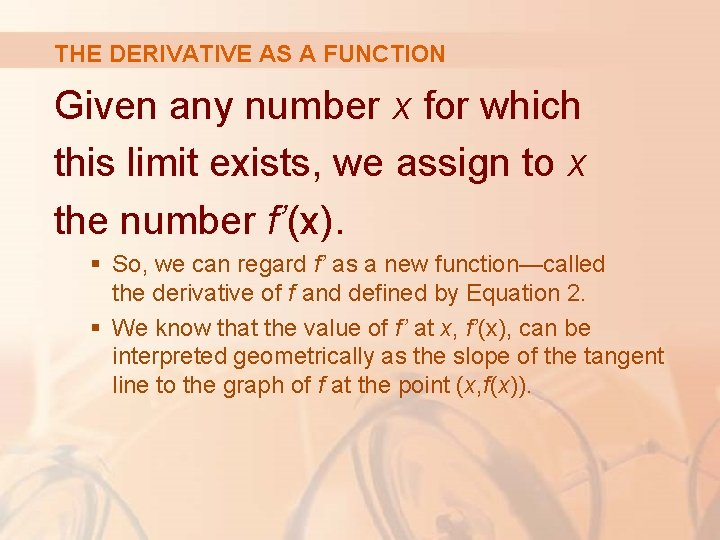THE DERIVATIVE AS A FUNCTION Given any number x for which this limit exists, we assign to x the number f’(x). § So, we can regard f’ as a new function—called the derivative of f and defined by Equation 2. § We know that the value of f’ at x, f’(x), can be interpreted geometrically as the slope of the tangent line to the graph of f at the point (x, f(x)).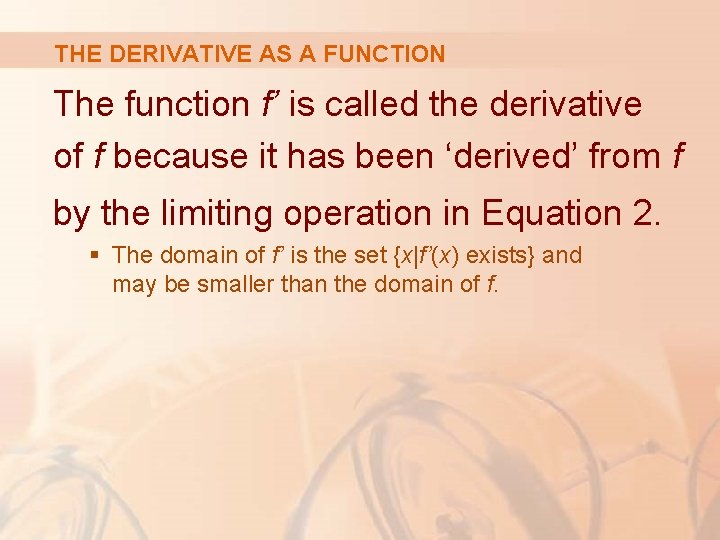THE DERIVATIVE AS A FUNCTION The function f’ is called the derivative of f because it has been ‘derived’ from f by the limiting operation in Equation 2. § The domain of f’ is the set {x|f’(x) exists} and may be smaller than the domain of f.THE DERIVATIVE AS A FUNCTION Example 1 The graph of a function f is given in the figure. Use it to sketch the graph of the derivative f’.THE DERIVATIVE AS A FUNCTION Example 1 We can estimate the value of the derivative at any value of x by drawing the tangent at the point (x, f(x)) and estimating its slope.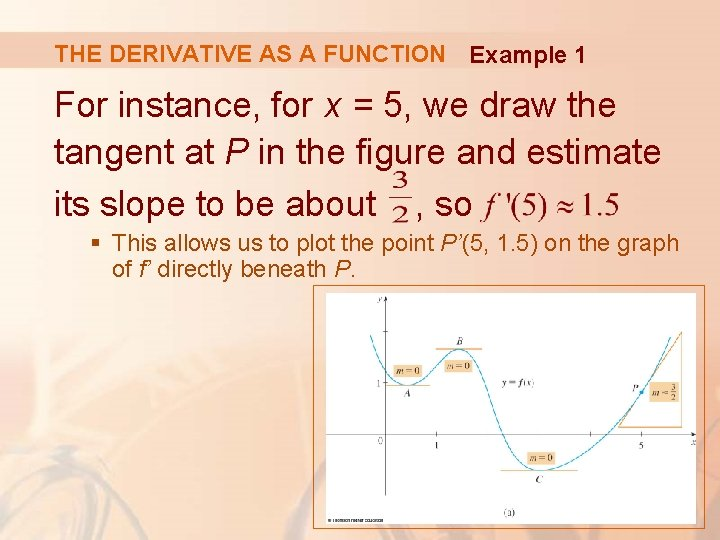THE DERIVATIVE AS A FUNCTION Example 1 For instance, for x = 5, we draw the tangent at P in the figure and estimate its slope to be about , so § This allows us to plot the point P’(5, 1. 5) on the graph of f’ directly beneath P.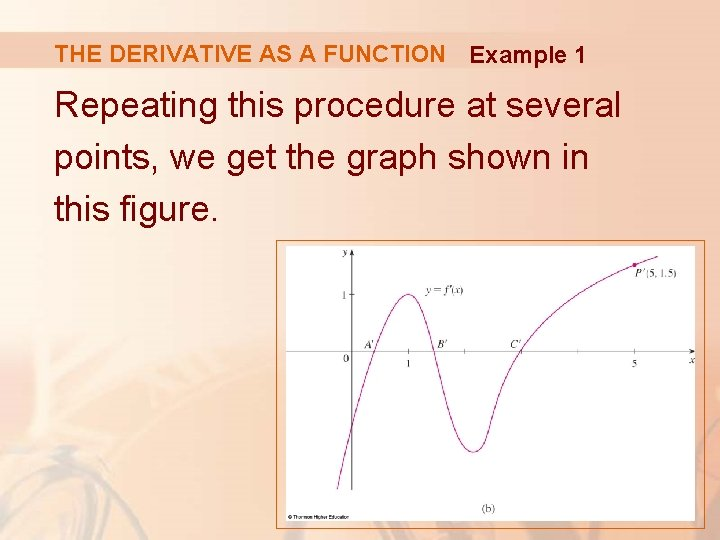THE DERIVATIVE AS A FUNCTION Example 1 Repeating this procedure at several points, we get the graph shown in this figure.THE DERIVATIVE AS A FUNCTION Example 1 Notice that the tangents at A, B, and C are horizontal. § So, the derivative is 0 there and the graph of f’ crosses the x-axis at the points A’, B’, and C’, directly beneath A, B, and C.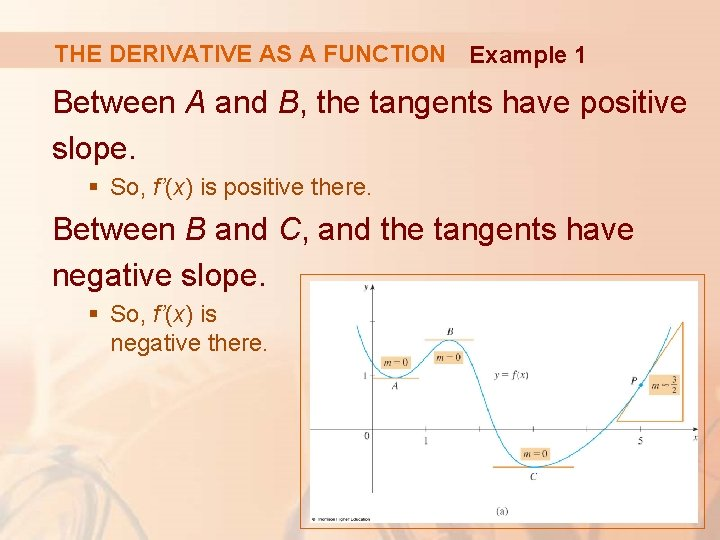THE DERIVATIVE AS A FUNCTION Example 1 Between A and B, the tangents have positive slope. § So, f’(x) is positive there. Between B and C, and the tangents have negative slope. § So, f’(x) is negative there.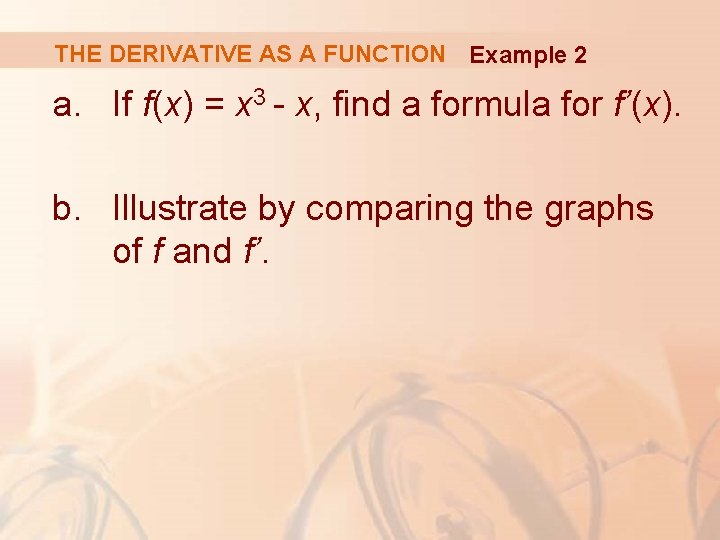THE DERIVATIVE AS A FUNCTION Example 2 a. If f(x) = x 3 - x, find a formula for f’(x). b. Illustrate by comparing the graphs of f and f’.THE DERIVATIVE AS A FUNCTION Example 2 a When using Equation 2 to compute a derivative, we must remember that: § The variable is h. § x is temporarily regarded as a constant during the calculation of the limit.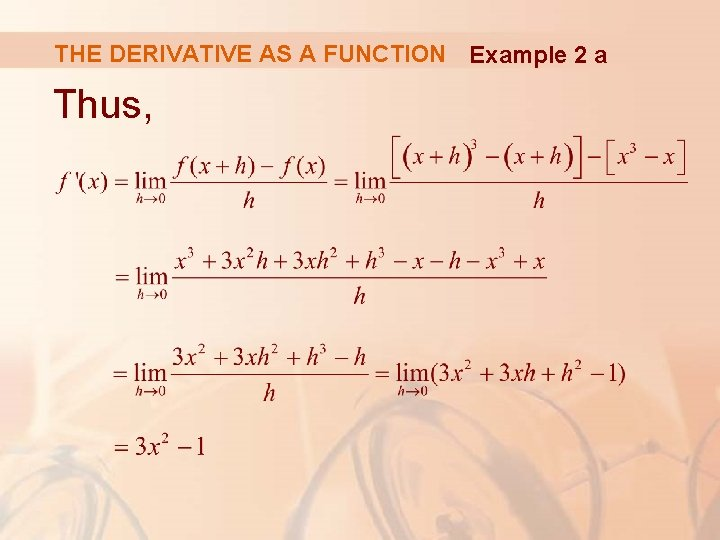THE DERIVATIVE AS A FUNCTION Example 2 a Thus,THE DERIVATIVE AS A FUNCTION Example 2 b We use a graphing device to graph f and f’ in the figure. § Notice that f’(x) = 0 when f has horizontal tangents and f’(x) is positive when the tangents have positive slope. § So, these graphs serve as a check on our work in part (a).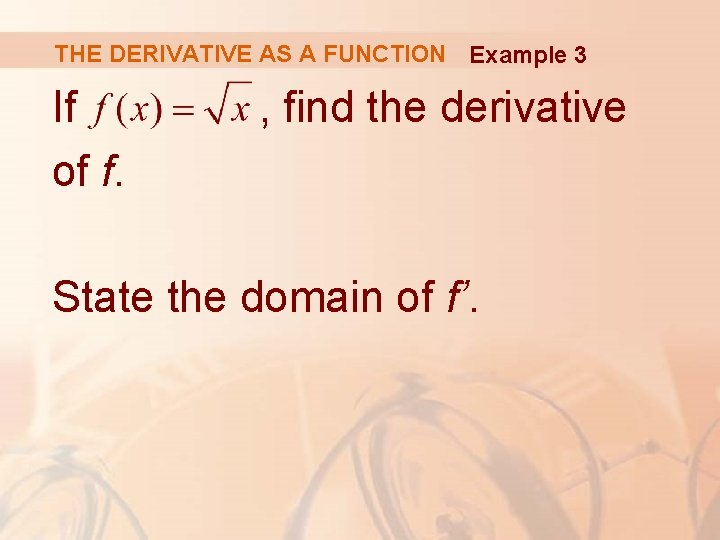THE DERIVATIVE AS A FUNCTION Example 3 If of f. , find the derivative State the domain of f’.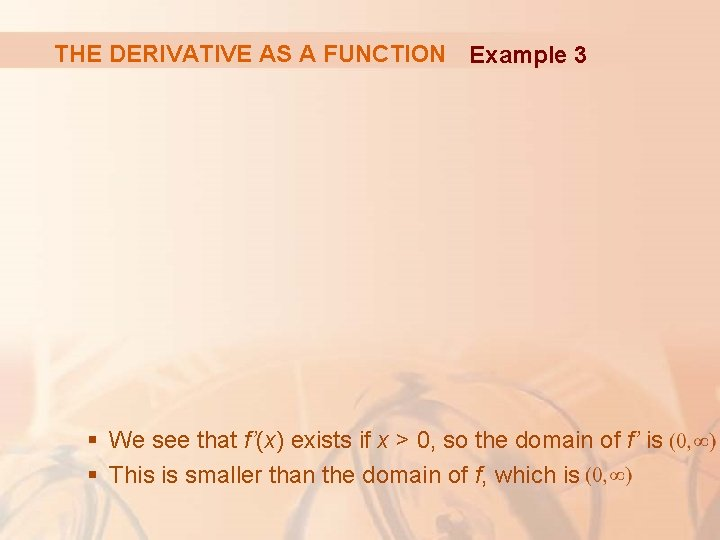THE DERIVATIVE AS A FUNCTION Example 3 § We see that f’(x) exists if x > 0, so the domain of f’ is § This is smaller than the domain of f, which isTHE DERIVATIVE AS A FUNCTION Let’s check to see that the result of Example 3 is reasonable by looking at the graphs of f and f’ in the figure.THE DERIVATIVE AS A FUNCTION When x is close to 0, is also close to 0. So, f’(x) = 1/(2 ) is very large. § This corresponds to the steep tangent lines near (0, 0) in (a) and the large values of f’(x) just to the right of 0 in (b).THE DERIVATIVE AS A FUNCTION When x is large, f’(x) is very small. § This corresponds to the flatter tangent lines at the far right of the graph of f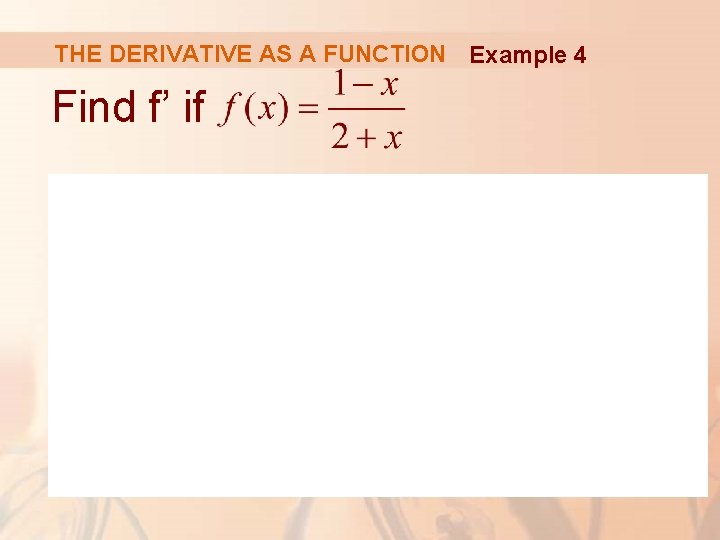THE DERIVATIVE AS A FUNCTION Example 4 Find f’ if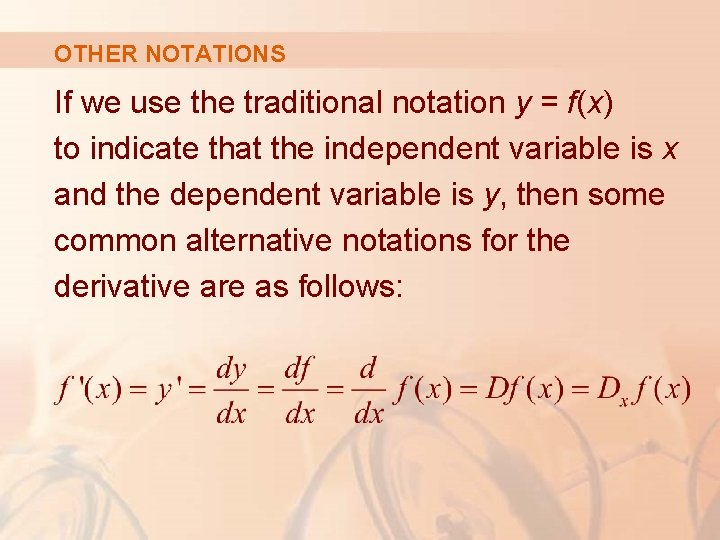OTHER NOTATIONS If we use the traditional notation y = f(x) to indicate that the independent variable is x and the dependent variable is y, then some common alternative notations for the derivative are as follows: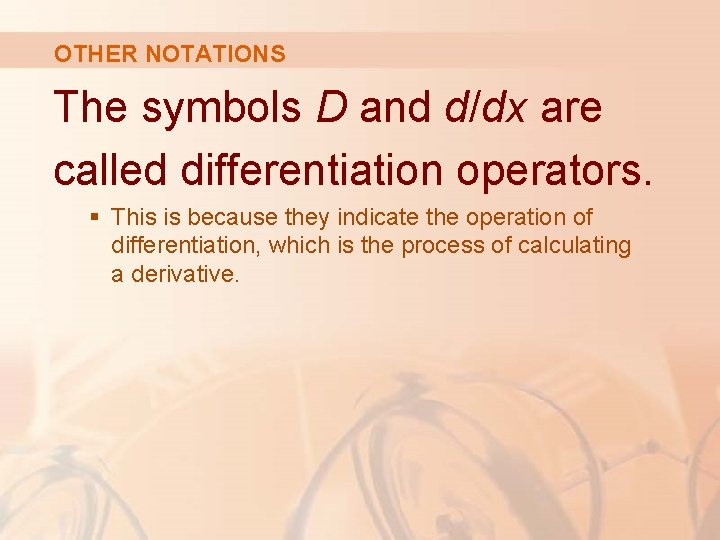OTHER NOTATIONS The symbols D and d/dx are called differentiation operators. § This is because they indicate the operation of differentiation, which is the process of calculating a derivative.OTHER NOTATIONS The symbol dy/dx—which was introduced by Leibniz—should not be regarded as a ratio (for the time being). § It is simply a synonym for f’(x). § Nonetheless, it is very useful and suggestive—especially when used in conjunction with increment notation.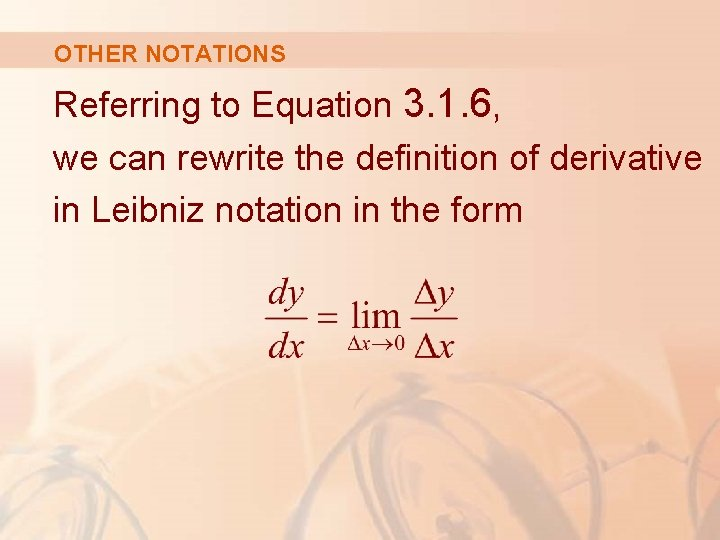OTHER NOTATIONS Referring to Equation 3. 1. 6, we can rewrite the definition of derivative in Leibniz notation in the formOTHER NOTATIONS If we want to indicate the value of a derivative dy/dx in Leibniz notation at a specific number a, we use the notation which is a synonym for f’(a).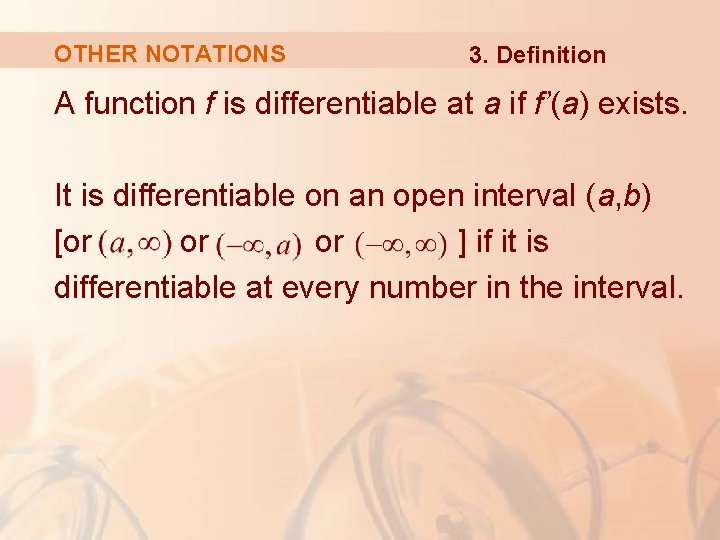OTHER NOTATIONS 3. Definition A function f is differentiable at a if f’(a) exists. It is differentiable on an open interval (a, b) [or or or ] if it is differentiable at every number in the interval.OTHER NOTATIONS Example 5 Where is the function f(x) = |x| differentiable? § If x > 0, then |x| = x and we can choose h small enough that x + h > 0 and hence |x + h| = x + h. § Therefore, for x > 0, we have: § So, f is differentiable for any x > 0.OTHER NOTATIONS Example 5 § Similarly, for x < 0, we have |x| = -x and h can be chosen small enough that x + h < 0 and so |x + h| = -(x + h). § Therefore, for x < 0, § So, f is differentiable for any x < 0.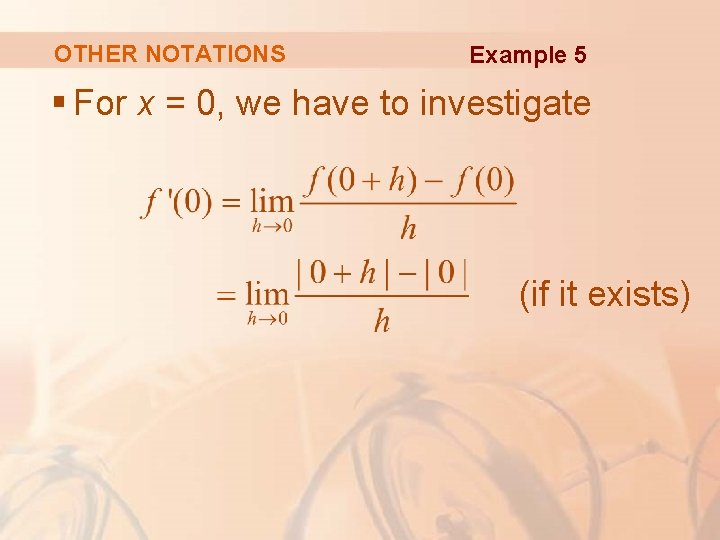OTHER NOTATIONS Example 5 § For x = 0, we have to investigate (if it exists)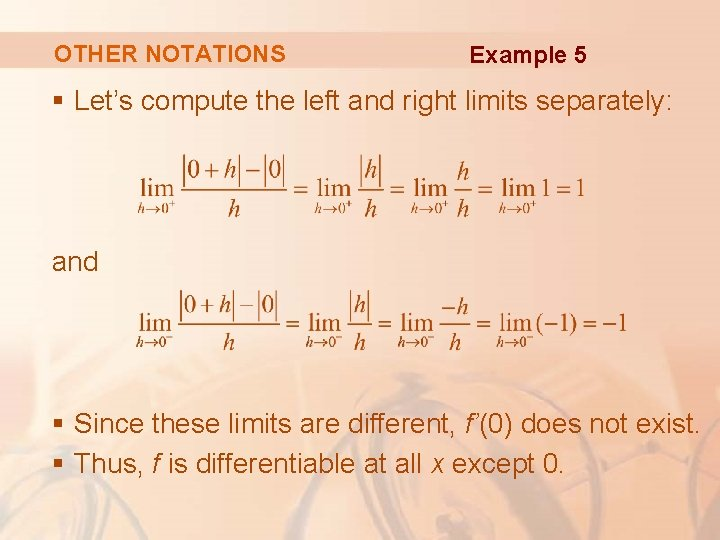OTHER NOTATIONS Example 5 § Let’s compute the left and right limits separately: and § Since these limits are different, f’(0) does not exist. § Thus, f is differentiable at all x except 0.OTHER NOTATIONS Example 5 A formula for f’ is given by: Its graph is shown in the figure.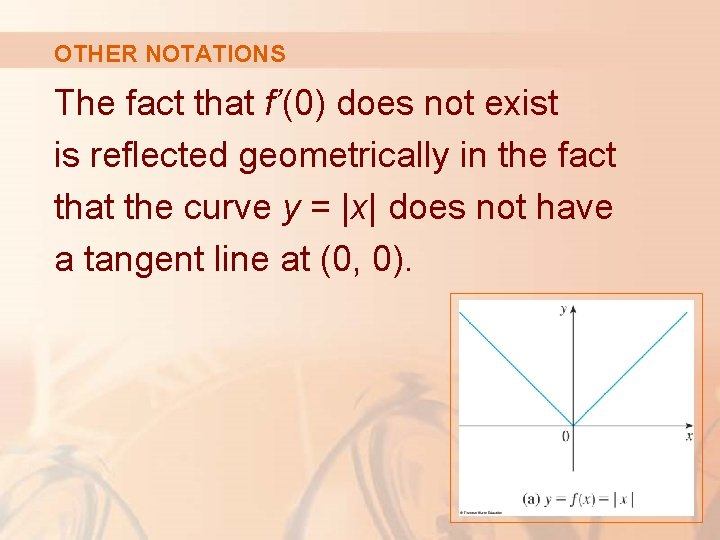OTHER NOTATIONS The fact that f’(0) does not exist is reflected geometrically in the fact that the curve y = |x| does not have a tangent line at (0, 0).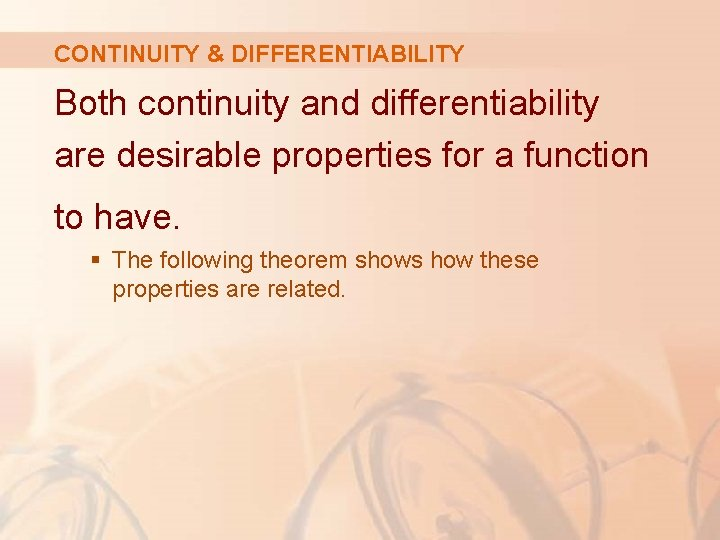CONTINUITY & DIFFERENTIABILITY Both continuity and differentiability are desirable properties for a function to have. § The following theorem shows how these properties are related.CONTINUITY & DIFFERENTIABILITY 4. Theorem If f is differentiable at a, then f is continuous at a. § To prove that f is continuous at a, we have to show that. § We do this by showing that the difference f(x) - f(a) approaches 0 as x approaches 0.CONTINUITY & DIFFERENTIABILITY Proof The given information is that f is differentiable at a. That is, § See Equation 3. 1. 5. exists.CONTINUITY & DIFFERENTIABILITY Proof To connect the given and the unknown, we divide and multiply f(x) - f(a) by x - a (which we can do when ):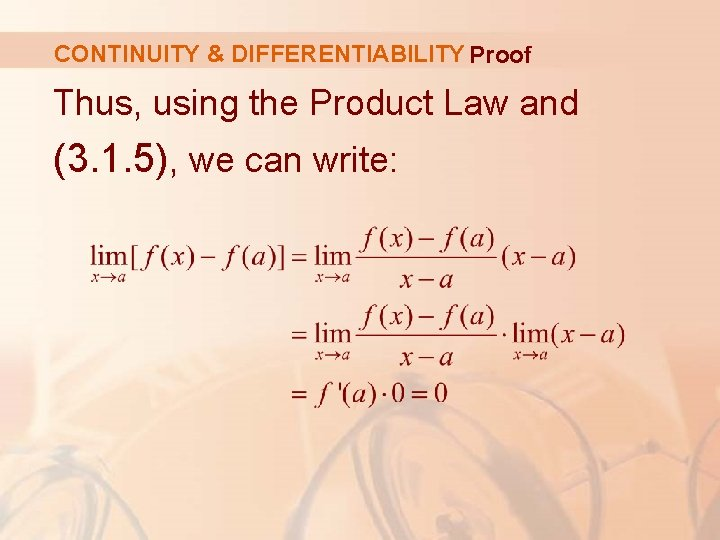CONTINUITY & DIFFERENTIABILITY Proof Thus, using the Product Law and (3. 1. 5), we can write: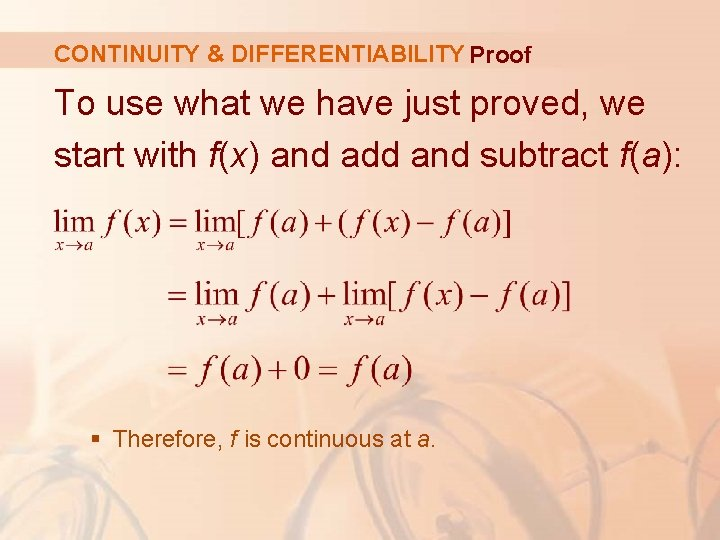CONTINUITY & DIFFERENTIABILITY Proof To use what we have just proved, we start with f(x) and add and subtract f(a): § Therefore, f is continuous at a.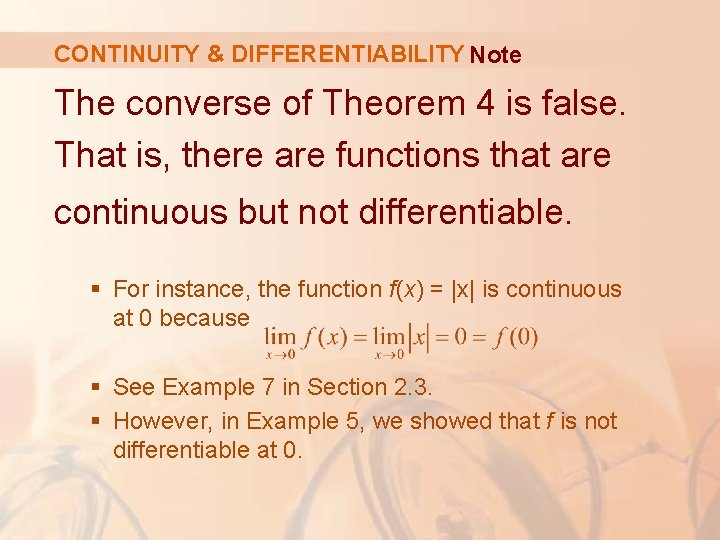CONTINUITY & DIFFERENTIABILITY Note The converse of Theorem 4 is false. That is, there are functions that are continuous but not differentiable. § For instance, the function f(x) = |x| is continuous at 0 because § See Example 7 in Section 2. 3. § However, in Example 5, we showed that f is not differentiable at 0.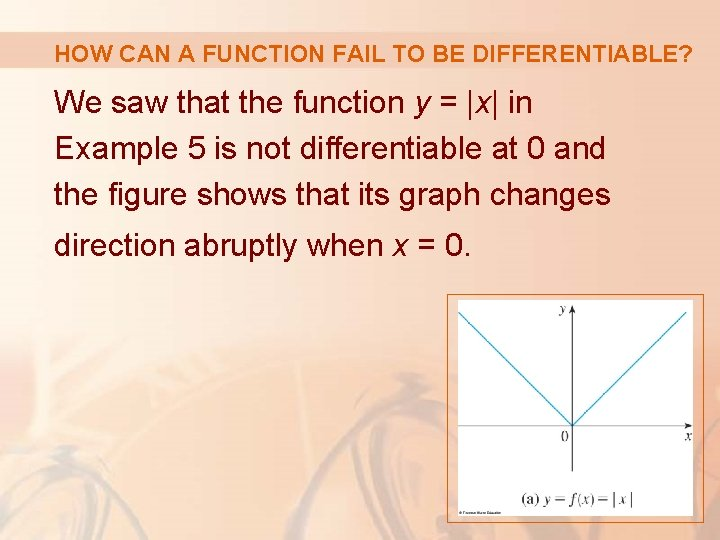HOW CAN A FUNCTION FAIL TO BE DIFFERENTIABLE? We saw that the function y = |x| in Example 5 is not differentiable at 0 and the figure shows that its graph changes direction abruptly when x = 0.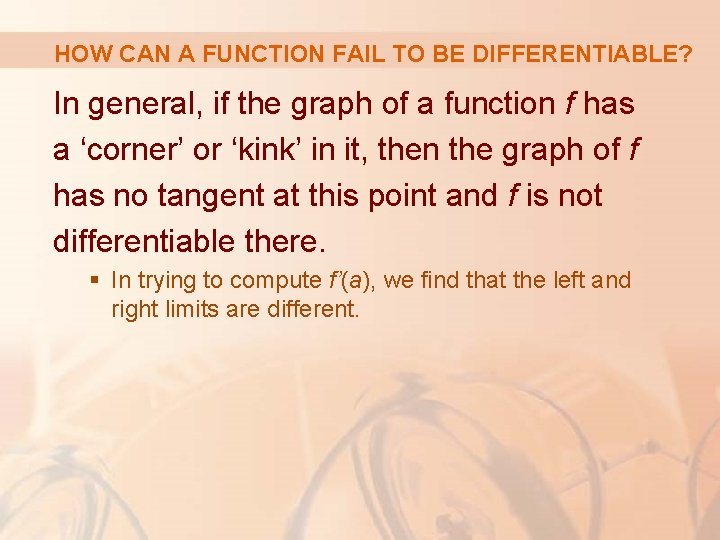HOW CAN A FUNCTION FAIL TO BE DIFFERENTIABLE? In general, if the graph of a function f has a ‘corner’ or ‘kink’ in it, then the graph of f has no tangent at this point and f is not differentiable there. § In trying to compute f’(a), we find that the left and right limits are different.HOW CAN A FUNCTION FAIL TO BE DIFFERENTIABLE? Theorem 4 gives another way for a function not to have a derivative. § It states that, if f is not continuous at a, then f is not differentiable at a. § So, at any discontinuity—for instance, a jump discontinuity—f fails to be differentiable.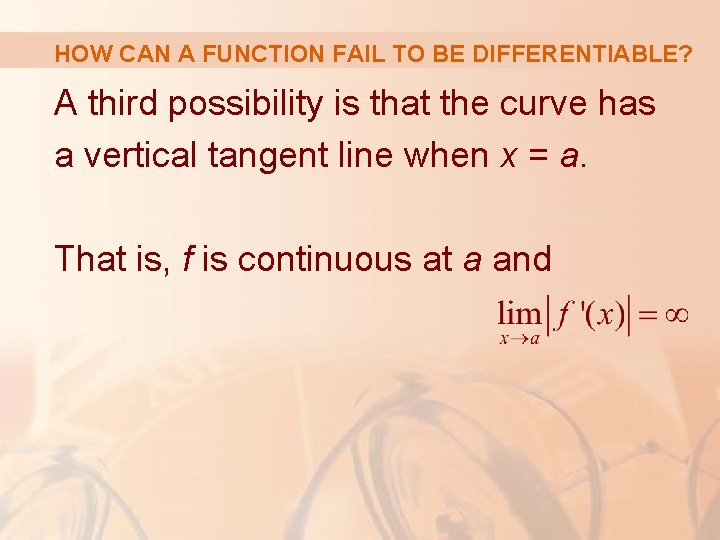HOW CAN A FUNCTION FAIL TO BE DIFFERENTIABLE? A third possibility is that the curve has a vertical tangent line when x = a. That is, f is continuous at a and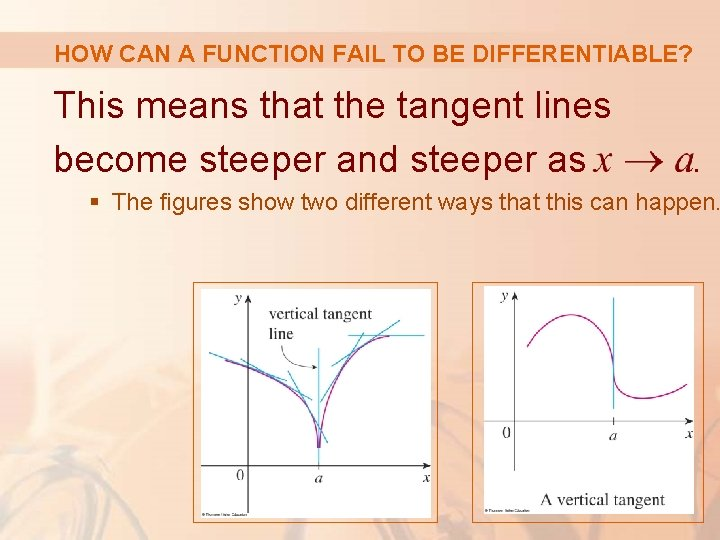HOW CAN A FUNCTION FAIL TO BE DIFFERENTIABLE? This means that the tangent lines become steeper and steeper as . § The figures show two different ways that this can happen.HOW CAN A FUNCTION FAIL TO BE DIFFERENTIABLE? The figure illustrates the three possibilities we have discussed.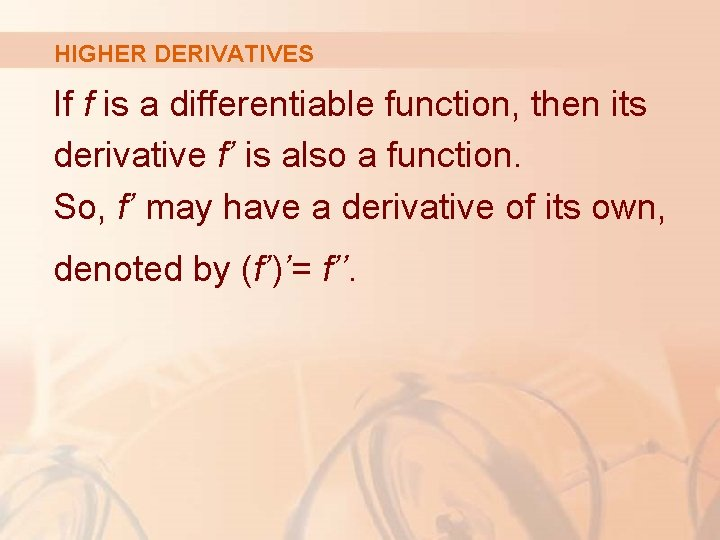HIGHER DERIVATIVES If f is a differentiable function, then its derivative f’ is also a function. So, f’ may have a derivative of its own, denoted by (f’)’= f’’.HIGHER DERIVATIVES This new function f’’ is called the second derivative of f. § This is because it is the derivative of f. § Using Leibniz notation, we write the second derivative of y = f(x) as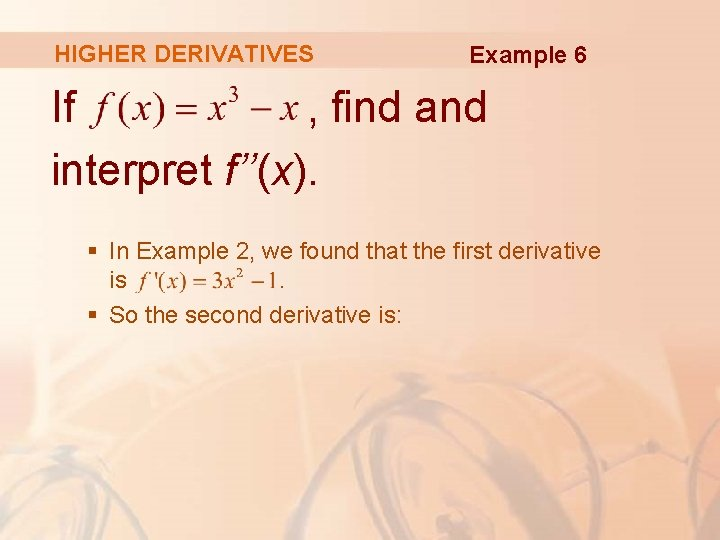HIGHER DERIVATIVES Example 6 If , find and interpret f’’(x). § In Example 2, we found that the first derivative is. § So the second derivative is:HIGHER DERIVATIVES Example 6 The graphs of f, f’’ are shown in the figure. § We can interpret f’’(x) as the slope of the curve y = f’(x) at the point (x, f’(x)). § In other words, it is the rate of change of the slope of the original curve y = f(x).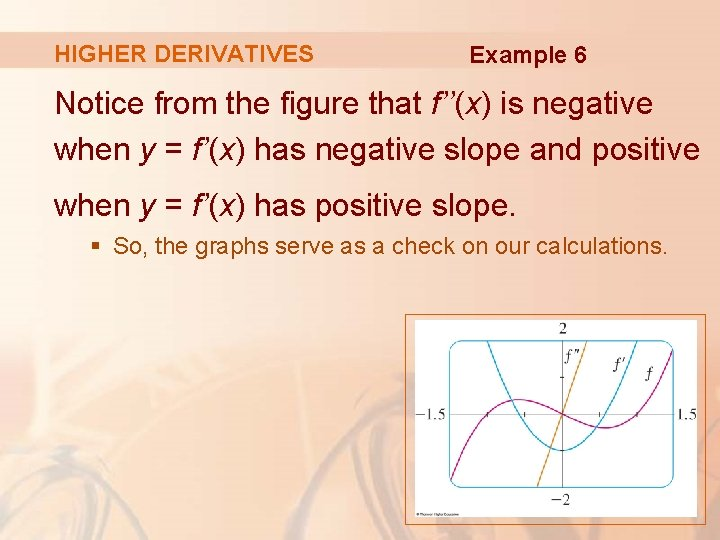HIGHER DERIVATIVES Example 6 Notice from the figure that f’’(x) is negative when y = f’(x) has negative slope and positive when y = f’(x) has positive slope. § So, the graphs serve as a check on our calculations.HIGHER DERIVATIVES In general, we can interpret a second derivative as a rate of change of a rate of change. § The most familiar example of this is acceleration, which we define as follows.HIGHER DERIVATIVES If s = s(t) is the position function of an object that moves in a straight line, we know that its first derivative represents the velocity v(t) of the object as a function of time: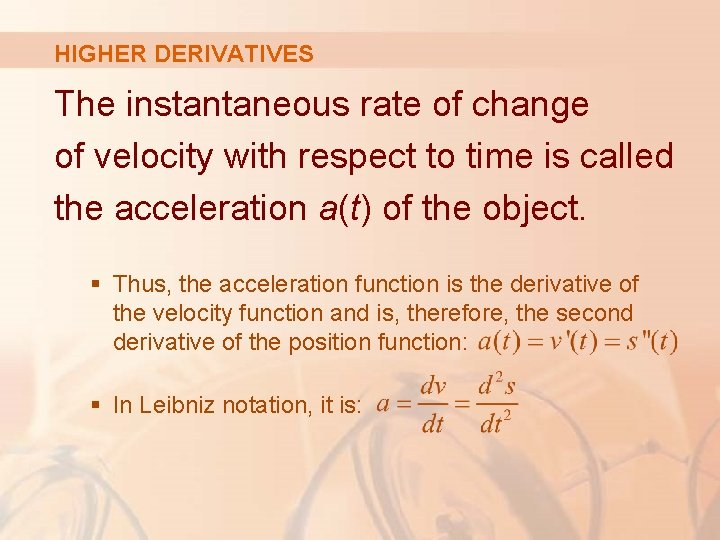HIGHER DERIVATIVES The instantaneous rate of change of velocity with respect to time is called the acceleration a(t) of the object. § Thus, the acceleration function is the derivative of the velocity function and is, therefore, the second derivative of the position function: § In Leibniz notation, it is: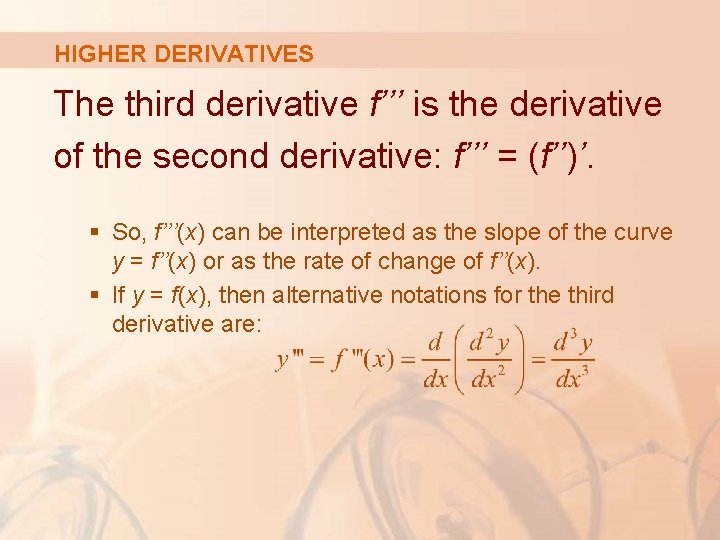HIGHER DERIVATIVES The third derivative f’’’ is the derivative of the second derivative: f’’’ = (f’’)’. § So, f’’’(x) can be interpreted as the slope of the curve y = f’’(x) or as the rate of change of f’’(x). § If y = f(x), then alternative notations for the third derivative are: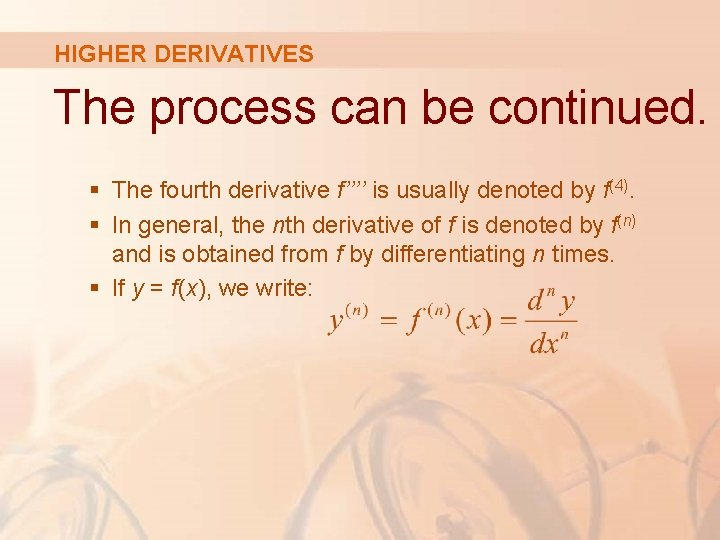HIGHER DERIVATIVES The process can be continued. § The fourth derivative f’’’’ is usually denoted by f(4). § In general, the nth derivative of f is denoted by f(n) and is obtained from f by differentiating n times. § If y = f(x), we write:HIGHER DERIVATIVES If (4) f (x). Example 7 , find f’’’(x) and § In Example 6, we found that f’’(x) = 6 x. § The graph of the second derivative has equation y = 6 x. § So, it is a straight line with slope 6.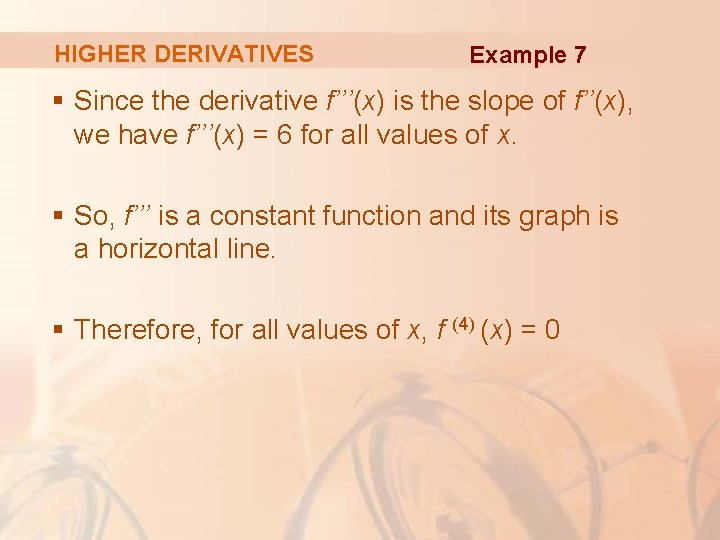HIGHER DERIVATIVES Example 7 § Since the derivative f’’’(x) is the slope of f’’(x), we have f’’’(x) = 6 for all values of x. § So, f’’’ is a constant function and its graph is a horizontal line. § Therefore, for all values of x, f (4) (x) = 0HIGHER DERIVATIVES We can interpret the third derivative physically in the case where the function is the position function s = s(t) of an object that moves along a straight line. § As s’’’ = (s’’)’ = a’, the third derivative of the position function is the derivative of the acceleration function. § It is called the jerk.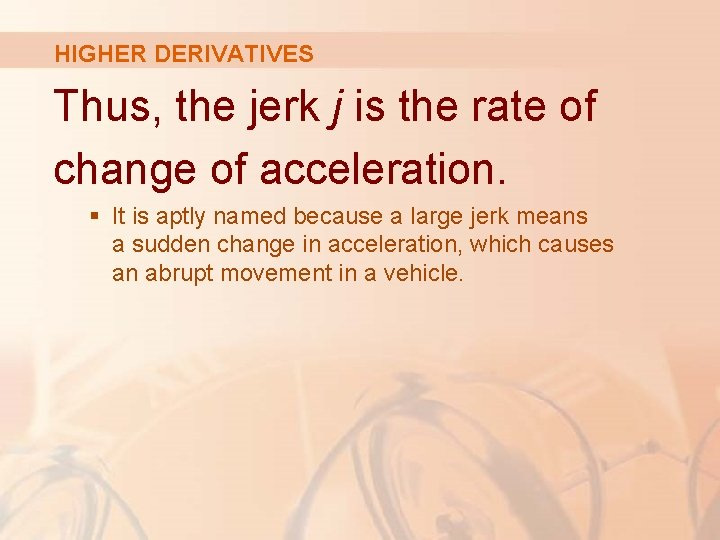HIGHER DERIVATIVES Thus, the jerk j is the rate of change of acceleration. § It is aptly named because a large jerk means a sudden change in acceleration, which causes an abrupt movement in a vehicle.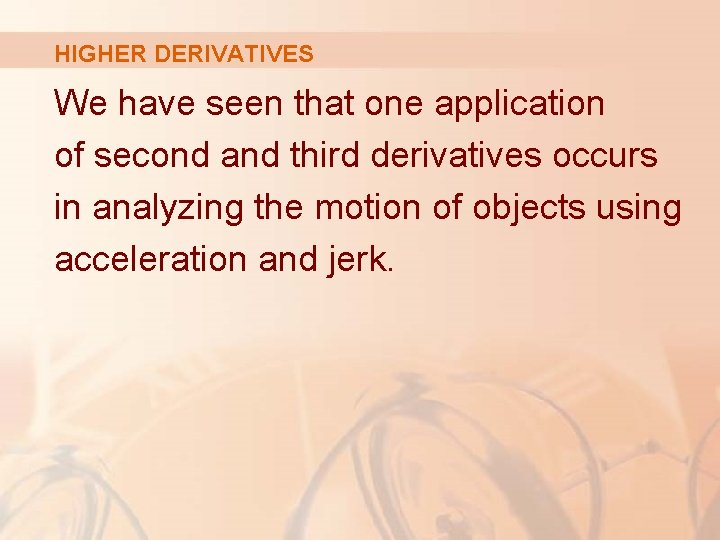HIGHER DERIVATIVES We have seen that one application of second and third derivatives occurs in analyzing the motion of objects using acceleration and jerk.